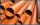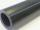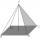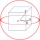# Metal tube

Calculate the metal tube mass 8dm long with the outer radius 5cm and the inner radius 4.5cm and 1cm3 of this metal is 9.5g.

m =  11.3411 kg

### Step-by-step explanation:Did you find an error or inaccuracy? Feel free to write us. Thank you!Tips to related online calculators
Do you know the volume and unit volume, and want to convert volume units?
Tip: Our Density units converter will help you with the conversion of density units.
Do you want to convert mass units?

#### You need to know the following knowledge to solve this word math problem:

We encourage you to watch this tutorial video on this math problem:

## Related math problems and questions:

• MassThe thickness of a metallic tube is 1cm, and its outer radius is 11cm. Find the mass of such a 1m long tube if the metal density is 7.5g per cubic cm.
• Steel tubeThe steel tube has an inner diameter of 4 cm and an outer diameter of 4.8 cm. The density of the steel is 7800 kg/m3. Calculate its length if it weighs 15 kg.
• Brass sphereFind the weight of a brass ball with an outer radius of 12 cm, a wall thickness of 20 mm if the brass's density is 8.5 g/cm3.
• The pipeThe pipe is 1.5 m long. Its outer diameter is 60 cm, inner diameter is 52 cm. Calculate the pipe's weight if the material's density from which it is made is 2 g/cm3. Round the results to whole kilograms.
• Iron densityCalculate the weight of a 2 m long rail pipe with an internal diameter of 10 cm and a wall thickness of 3 mm. The iron density is p = 7.8 g/cm3.
• The castThe cast in the body of a regular quadrilateral pyramid with a base edge 60 cm long and 5 cm high is made of a material with a density of 7.8 g/cm cubic. Calculate its weight.
• Metal pyramidFind the weight of a regular quadrilateral pyramid with a 5 cm length and 6,5 cm body height is made from material with density g/cm3.
• Brass ballWhat is the weight of a hollow brass ball, the outer diameter of which is 16 cm and the wall thickness is 2 cm, while the density of brass is ϱ = 8.5 g
• The hollow cylinderThe hollow cylinder has a height of 70 cm, an outer diameter of 180 cm, and an inner diameter of 120 cm. What is the surface of the body, including the area inside the cavity?
• Cuboid 5Calculate the mass of the cuboid with dimensions of 12 cm; 0.8 dm and 100 mm made from spruce wood (density = 550 kg/m3).
• Cylinder - hThe cylinder volume is 140 cm3. The base radius is 7 cm. Calculate the height of the cylinder.
• LidWhat is the weight of concrete cover (lid) to round shape well with a diameter 1.8 m, if the thickness of the cover is 11 cm? 1 m3 of concrete weighs 2190 kg.
• AL wireWhat is the weight of an aluminum wire 250 m long with a diameter of 2 mm, if the density of aluminum is p = 2700 kg/m cubic. Determine to the nearest gram.
• Brass tubeThe outer perimeter of brass tube (ρ = 8.5 g/cm3) is 38 cm. Its mass is 5 kg, length 54 cm. What is the pipe wall thickness?
• Oak trunkCalculate in tonnes the approximate weight of a cylindrical oak trunk with a diameter of 66 cm and a length of 4 m, knowing that the density of the wood was 800 kg/m³.
• Cylinder - h2Cylinder volume is 2.6 liters. Base area is 1.3 dm2. Calculate the height of the cylinder.
• The bodyThe body has dimensions of 2m, 2dm, and 10 cm. It weighs 28 kg. What is its density?